### Home > PC > Chapter 1 > Lesson 1.4.2 > Problem1-148

1-148.
1. Find the value of x in each triangle below. Homework Help ✎

1.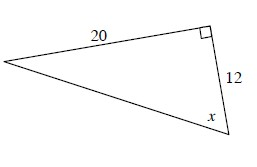2.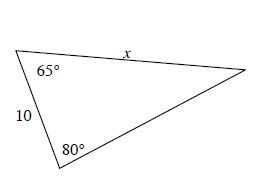3.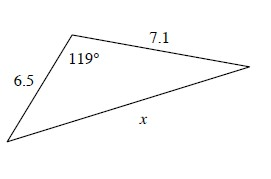4.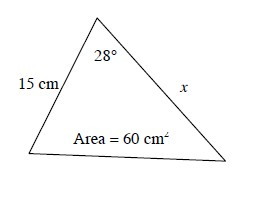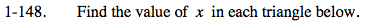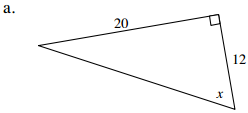Set up a ratio using the tangent function.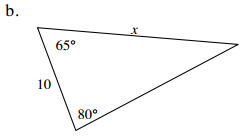Find the 3rd angle. Then use the Law of Sines.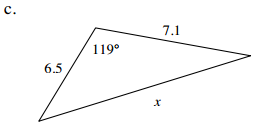You do not have an angle with an opposite side. So use the Law of Cosines.

≈ 11.72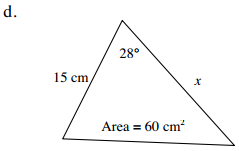Drop a perpendicular line from angle F to AC.

Using the sine function, find h in terms of the sin 28° and x.

Set the area

$(60) = \frac{1}{2}\text{(base)(height)}$

≈ 17.04 cm